#### Howdy, Stranger!

We are about to switch to a new forum software. Until then we have removed the registration on this forum.

• i have a research paper i have to implement this paper,please anyone help me out to write codes

I'm guessing this involves ASCII format bulk data, but @varundubey9935 never posts more than a single sentence, so it is really hard to know.

Did you try toxiclibs, and did it work for you? Can you load data? Can you make a mesh? You should tell us -- I have no idea, so I can't help you.

For STL in Processing, use the toxiclibs STLReader and STLWriter.

Here is their test code for using it. Be sure that you understand the STL file format.

Please, no duplicates! If you want people to answer your question, post it once, and comment on it to update the question or add more detail. Posting tiny bits of the same question several places and ignoring answers makes people frustrated and unlikely to help you in the future.

Please, no duplicates as it is hard to keep track of the same posts. Consider checking https://forum.processing.org/two/search?Search=STLReader

Kf

• Import STL

Addendum: The toxiclibs STLReader and STLWriter classes are a bit more complex than I thought, but not much -- and they have test code for using it as well. Whether taking it on yourself or just submitting a p5.js feature request, they are a good place to start for adding STL support to p5.js.

• Initial position the object with Proscene in Processing

Hello guys,

I'm trying to combine the use of toxilibs and proscene libraries to view objects in a scene.

How could I set the initial positions of the object? (More to the middle of the screen, and a certain angle - straight for example).

I'm trying to combine more than one command, but it looks like a interfefe on the other, one could give any tips?

Below is the code and the image, to pass a better idea I'm trying to do.

Note: You need a .stl file to render and libraries, of course ...

Code import toxi.geom.*; import toxi.geom.mesh.*; import toxi.processing.*; import remixlab.proscene.*; import remixlab.dandelion.core.*; import remixlab.dandelion.geom.*;

``````TriangleMesh mesh;
ToxiclibsSupport gfx;

Scene scene;
Trackable lastAvatar;
Particle[] particle;

float p1 = -1, p2 = -50, p3 = 30, p4 = -15;
float zoom         = 7;
int nbPart         = 2000;
boolean avoidWalls = true;
//int flockWidth = 1280, flockHeight = 720, flockDepth = 600;

PVector pCam;

void setup() {
size(displayWidth, displayHeight, OPENGL);
surface.setTitle("Corpo Humano");
noStroke();
//smooth();
//lights(); //Inicia Luzes

pCam = new PVector(-1, -50, 30);

//Inicia ambiente para Cena
scene = new Scene(this);
scene.setAxesVisualHint(false);
scene.setGridVisualHint(false);
scene.setAnimationPeriod(40); // 25Hz
scene.mouseAgent().setPickingMode(MouseAgent.PickingMode.CLICK);

//scene.setBoundingBox(new Vec(0, 0, 0), new Vec(flockWidth, flockHeight, flockDepth)); //
//scene.camera().setTranslationSensitivity(-10);
//scene.camera().frame().setRotationSensitivity(1.5);
//scene.camera().setUpVector(new Vec(0, 0, -1), true); // boolean noMove

scene.camera().setPosition(new Vec(10, -90, -25));
scene.camera().lookAt(new Vec(10, 30, 30));
//scene.camera().frame().setRotation(p1, p2, p3, p4);

scene.showAll();
scene.startAnimation();

gfx  = new ToxiclibsSupport(this); //Objeto para atualizar "desenho"

particle = new Particle[nbPart];
for (int i = 0; i < particle.length; i++)
particle[i] = new Particle();
}

void draw() {
background(37);

//Efeitos de Luz na Cena
ambientLight(10, 10, 120);
directionalLight(192, 168, 128,0, -1000, -0.5);
directionalLight(255, 64, 0, 0.5f, -0.5f, -0.1f);

scale(zoom);         //Muda scala para zoom
gfx.mesh(mesh,true); //Desenha Objeto

pushStyle();
strokeWeight(0.7*-1);
beginShape(POINTS);
for (int i = 0; i < nbPart; i++) {
particle[i].draw();
}
endShape();
popStyle();
}

void keyPressed() {
//validaTecla(key);

//Teste
if (key == '1') p1 += 1.5;
else if (key == '2') p1 -= 1.05;
else if (key == '3') p2 += 1.05;
else if (key == '4') p2 -= 1.05;
else if (key == '5') p3 += 1.05;
else if (key == '6') p3 -= 1.05;
else if (key == '7') p4 += 1.05;
else if (key == '8') p4 -= 1.05;

scene.camera().frame().setRotation(p1, p2, p3, p4);
println("Pontos: " + p1, p2, p3, p4);
}

void mousePressed(){
//println("Camera: " + scene.camera().getPosition(); ??
}

if (scene.avatar() != null)
frameRate(1000/scene.animationPeriod());
else
frameRate(60);

if (scene.animationStarted())
scene.restartAnimation();
}

void animateScene(Scene s) {
for (int i = 0; i < nbPart; i++)
if (particle[i] != null)
particle[i].animate();
}

class Particle {
PVector speed;
PVector pos;
int age;
int ageMax;

public Particle() {
speed = new PVector();
pos = new PVector();
init();
}

public void animate() {
speed.z -= 0.05f;

if (pos.z < 0.0) {
speed.z = -0.8f * speed.z;
pos.z = 0.0f;
}

if (++age == ageMax)
init();
}

public void draw() {
strokeWeight(0.2);
stroke( 255 * ((float) age / (float) ageMax), 255 * ((float) age / (float) ageMax), 255);
vertex(pos.x, pos.y, pos.z);
}

public void init() {
pos = new PVector(0.0f, 0.0f, 0.0f);
float angle = 2.0f * PI * random(1);
float norm = 0.04f * random(1);
speed = new PVector(norm * cos(angle), norm * sin(angle), random(1));
age = 0;
ageMax = 50 + (int) random(100);
}
}
``````

Initial position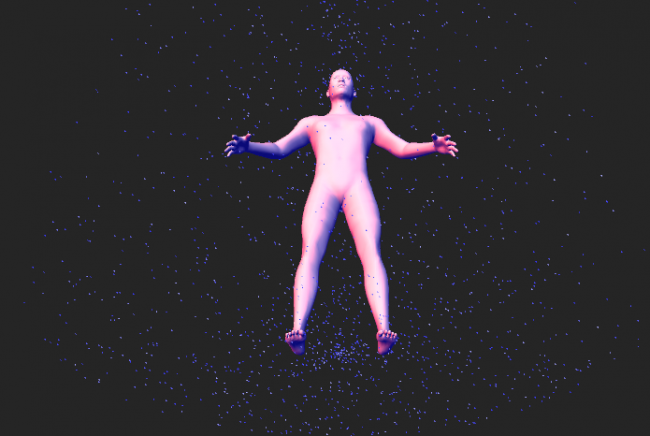• [toxiclibs] Import Toxi Mesh from STL in Android Mode

I cant seem to import a mesh as a toxi mesh.

``````import toxi.geom.*;
import toxi.geom.mesh.*;
import toxi.processing.*;
import toxi.geom.mesh.TriangleMesh;
import toxi.processing.ToxiclibsSupport;

import processing.opengl.*;

ToxiclibsSupport gfx;
TriangleMesh cave;

void setup() {
fullScreen(P3D);
gfx = new ToxiclibsSupport(this);
}

void draw() {

background(0);
camera(0, 0, 10, 0, 0, -50, 0, -1, 0);
directionalLight(255, 64, 0, 0.5f, -0.1f, 0.5f);
fill(255);
noStroke();
gfx.mesh(cave, false);
}
``````

It compiles but it crashes on the phone.

This one however works

``````import toxi.geom.*;
import toxi.geom.mesh.*;
import toxi.math.*;
import toxi.processing.*;

import processing.opengl.*;

float NOISE_SCALE = 0.08f;
int DIM=40;

Terrain terrain;
ToxiclibsSupport gfx;
Mesh3D mesh;

void setup() {
fullScreen(P3D);
terrain = new Terrain(DIM, DIM, 50);
float[] el = new float[DIM*DIM];
noiseSeed(23);
for (int z = 0, i = 0; z < DIM; z++) {
for (int x = 0; x < DIM; x++) {
el[i++] = noise(x * NOISE_SCALE, z * NOISE_SCALE) * 400;
}
}
terrain.setElevation(el);
mesh = terrain.toMesh();
gfx = new ToxiclibsSupport(this);
}

void draw() {
background(0);
camera(0, 0, 10, 0, 0, -50, 0, -1, 0);
directionalLight(255, 64, 0, 0.5f, -0.1f, 0.5f);
fill(255);
noStroke();
gfx.mesh(mesh, false);
}
``````

It looks like toxiclib works in processing for android so I'm not sure why I'm getting this problem.

• Drawing the human silhouette with bezier

Hello guys,

I'm leaving the solution I found here, because it can be helpful to others ...

I was "another way" to "build" the human being, I used MakeHuman Application http://www.makehuman.org/

The model can export to .stl Using toxilibs library, you can download and view the image from the .stl file

To zoom and rotation, used the peaseCam library ...

Below is the code to run must have a file in the folder .stl type of course ...

Note: It can be enhanced with 3D software like Blender for example, but this is another story ... well, what to do from the this, will the goals you want ...

Thank you,

Code:

``````        import toxi.geom.*;
import toxi.geom.mesh.*;
import toxi.processing.*;
import peasy.*;

TriangleMesh mesh;
ToxiclibsSupport gfx;
PeasyCam cam;

int zoom = 30;

void setup() {
size(displayWidth, displayHeight, P3D);

gfx  = new ToxiclibsSupport(this);

cam = new PeasyCam(this, 800);
cam.setMinimumDistance(10);
cam.setMaximumDistance(800);

noStroke();
}
void draw() {
background(0);
rotateX(-.2);
rotateY(-.2);

pushMatrix();
translate(0,0,20);
directionalLight(192, 168, 128,0, -1000, -0.5);
directionalLight(255, 64, 0, 0.5f, -0.5f, -0.1f);
scale(zoom);
gfx.mesh(mesh,false);
popMatrix();
}

void keyPressed(){
if (key==CODED) {
if (keyCode == UP) zoom++;
else if(keyCode == DOWN) zoom--;
}
}
``````

Image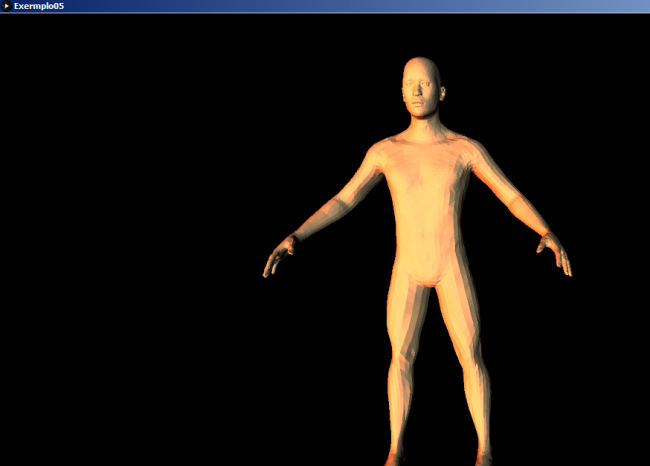• SOLVED [Toxiclibs] Is there a quicker method to test mesh inclusion

Hi

I was looking to see if I could test if a point was inside a mesh. I was looking online and found this article which suggested raytracing as a method and find the number of intersections. Using toxiclibs I could find if a ray intersected a mesh only according to the mesh normals. So I had to check against two meshes, one which is flipped.

It works but it just takes a long time to test and I also have to loop through the function a few times to get rid of all the points( even though I shouldnt need to).

Anyway is there a quicker method to check that, maybe with another library. Here is my code.

``````import java.util.*;
import peasy.*;
import toxi.geom.*;
import toxi.geom.mesh.*;
import toxi.processing.ToxiclibsSupport;

import wblut.processing.*;
import wblut.hemesh.*;
import wblut.geom.*;

private ToxiclibsSupport gfx;
TriangleMesh cave;
TriangleMesh cave2;

ArrayList<Vec3D> pts = new ArrayList<Vec3D>();

public void settings() {
size(1400, 800, P3D);
smooth();
}

public void setup() {

cave2.flipVertexOrder();

Vec3D a = cave.computeCentroid();
PeasyCam cam = new PeasyCam(this, a.x, a.y, 0, 2200);

gfx = new ToxiclibsSupport(this);

for (int i = 0; i < 20; i++) {
for (int j = 0; j < 20; j++) {
for (int k = 0; k < 20; k++) {
pts.add(new Vec3D(i * 70, j * 70, k * 30));
}
}
}

//Point in Mesh Function ( a bit slow)

for (int j = 0; j < 10; j++) {    // Need to run it a few times
for (int i = 0; i < pts.size(); i++) {
Vec3D v = pts.get(i);
Ray3D r = new Ray3D(v, new Vec3D(0, 0, 1));
if (!cave.intersectsRay(r)) {
pts.remove(v);
} else {
if (cave2.intersectsRay(r)) {
pts.remove(v);
}
}
}
}

}

public void draw() {
background(0);

for (Vec3D a : pts) {
stroke(255);
strokeWeight(2);
point(a.x, a.y, a.z);
}

pushMatrix();
fill(40, 120);
noStroke();
lights();
gfx.mesh(cave2, false, 0);
popMatrix();

}
``````
• How to call an instance of an object in a new class in an IDE?

I was wondering how I would be able to call an instance of a class from the main sketch in on of the classes i have created.

For example here how would I call the instance of WETriangleMesh "cave" in the new class meshpts. I have put notes where I am getting the error.

I have tried to run this in both IntelliJ and Eclipse but I can,t seem to figure it out.

Root Sketch

``````import processing.core.PApplet;
import peasy.*;
import toxi.geom.mesh.*;
import toxi.processing.ToxiclibsSupport;

public class MainApp extends PApplet {

PeasyCam cam;
ToxiclibsSupport gfx;
WETriangleMesh cave;
Meshpts pts;

public static void main(String[] args){
PApplet.main("MainApp", args);
}

public void settings(){
size(1400, 800,P3D);
smooth();
}

public void setup(){
cam = new PeasyCam(this,750,750,0,2200);
pts = new Meshpts(this);
gfx=new ToxiclibsSupport(this);
}

public void draw(){
background(0);

stroke(255, 0, 0);
noFill();

cave.run();

pushMatrix();
fill(40, 120);
noStroke();
strokeWeight(1);
stroke(10);
lights();
gfx.mesh(cave, false, 10);
popMatrix();

}
}
``````

Class Meshpts

``````import processing.core.PApplet;

public class Meshpts{
PApplet p;

public Meshpts(PApplet p){
this.p = p;
}

void run() {
cave.computeCentroid(); // error is here
}

}
``````
• Size Issue After STL image import

I wrote a program in Processing, to import a STL file and then rotate in 3D according to requirement. But I am facing a problem that the import image is very diminish in size.

``````import toxi.geom.*;
import toxi.geom.mesh.*;

import toxi.processing.*;

TriangleMesh mesh;
ToxiclibsSupport gfx;

void setup() {
size(displayWidth, displayHeight,P3D);
gfx=new ToxiclibsSupport(this);
}

void draw() {
background(51);
translate(width/2,height/2,0);
rotateX(mouseY*0.01);
rotateY(mouseX*0.01);

gfx.mesh(mesh,false,10);
}
``````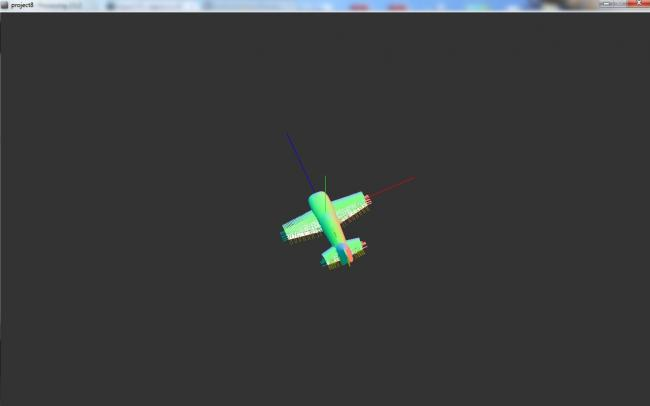• import 3D image in Processing

Thank you @KevinWorkman I checked last link and it is working

``````import toxi.geom.*;
import toxi.geom.mesh.*;

import toxi.processing.*;

TriangleMesh mesh;
ToxiclibsSupport gfx;

void setup() {
size(600,600,P3D);
gfx=new ToxiclibsSupport(this);
}

void draw() {
background(51);
lights();
translate(width/2,height/2,0);
rotateX(mouseY*0.01);
rotateY(mouseX*0.01);
gfx.origin(new Vec3D(),200);
noStroke();
gfx.mesh(mesh,false,10);
}
``````
• Import STL file using Toxiclibs

I am using Toxiclibs and I can successfully import STL file but something goes wrong. As you can see the pic, I didn't add any colorful things in the model. Can somebody tell me what is wrong?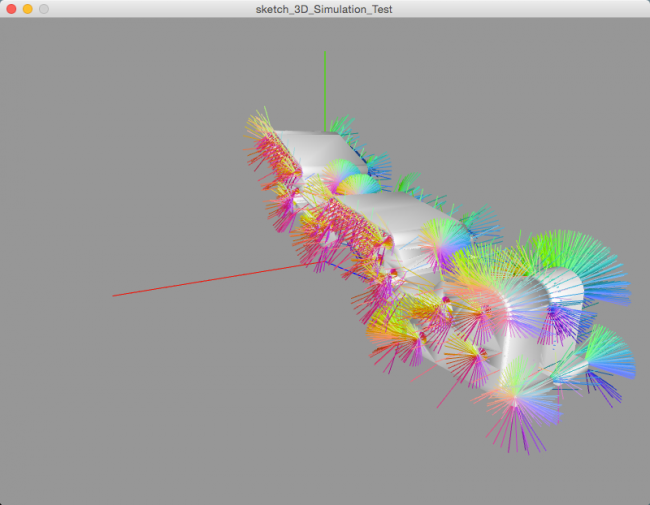import processing.core.PApplet; import toxi.geom.Vec3D; import toxi.geom.mesh.STLReader; import toxi.geom.mesh.TriangleMesh; import toxi.processing.ToxiclibsSupport;

``````ToxiclibsSupport gfx;
TriangleMesh mesh;

public void setup()
{
size( 800, 600, P3D);
gfx = new ToxiclibsSupport(this);
}

public void draw()
{
background(151);
lights();
translate(width/2, height/2, 300);
rotateX(mouseY*0.01f);
rotateY(mouseX*0.01f);
gfx.origin(new Vec3D(), 100);
noStroke();
gfx.mesh(mesh, true, 10);
}
``````
• [toxiclibs] intersect mesh with ray

It seems to me that intersection ray with mesh does not works.

This is my code:

``````import javax.print.attribute.standard.PresentationDirection;

import peasy.PeasyCam;
import processing.core.PApplet;
import toxi.geom.AABB;
import toxi.geom.IsectData3D;
import toxi.geom.Ray3D;
import toxi.geom.Vec3D;
import toxi.geom.mesh.TriangleMesh;
import toxi.physics.VerletParticle;
import toxi.physics.VerletPhysics;
import toxi.physics.VerletSpring;
import toxi.physics.behaviors.GravityBehavior;
import toxi.physics.constraints.BoxConstraint;
import toxi.processing.ToxiclibsSupport;

public class RayMeshIntersectionTest extends PApplet {

public static void main(String[] args) {
PApplet.main(new String[] { "RayMeshIntersectionTest" });
}

PeasyCam cam;
ToxiclibsSupport gfx;

TriangleMesh meshSTL;
Ray3D ray;

final int MOVE_RAY = 0;
final int MOVE_CAM = 1;
private int mode = MOVE_CAM;

public void setup()
{
size( 960, 720, P3D);
gfx = new ToxiclibsSupport(this);
cam = new PeasyCam(this, 400);
cam.setMinimumDistance(50);
cam.setMaximumDistance(1500);

meshSTL.scale(100);

ray = new Ray3D(new Vec3D(0,0,-200), new Vec3D(0,0,1));

}

public void draw()
{
update();

background(151);
lights();
directionalLight(255,255,255,-500,200,300);
specular(255);
shininess(32);

gfx.origin(new Vec3D(), 100);

stroke(255,255,0);
noFill();
gfx.mesh(meshSTL, true, 0);

fill(255,0,0);
stroke(255,0,0);
gfx.ray(ray, 400);
}

private void update() {
if(mode == MOVE_RAY) {
ray.setX(mouseX - width/2);
ray.setY(mouseY - height/2);
}

System.out.println( meshSTL.intersectsRay(ray) );
IsectData3D isec = meshSTL.getIntersectionData();
System.out.println(isec.pos);
}

public void keyPressed() {
if(key==' '){
mode = MOVE_RAY;
}
}

public void keyReleased() {
mode = MOVE_CAM;
}
}
``````

isec.pos is always null

• [toxiclibs] import 3D object

Hi! I'm trying to import a 3d object into processing. I have the obj file (and exported in stl binary format with Rhino) and I'm trying to import it into a toxic lib triangle mesh. What I get is not What I'm expecting.

Here is my object seen in Rhino: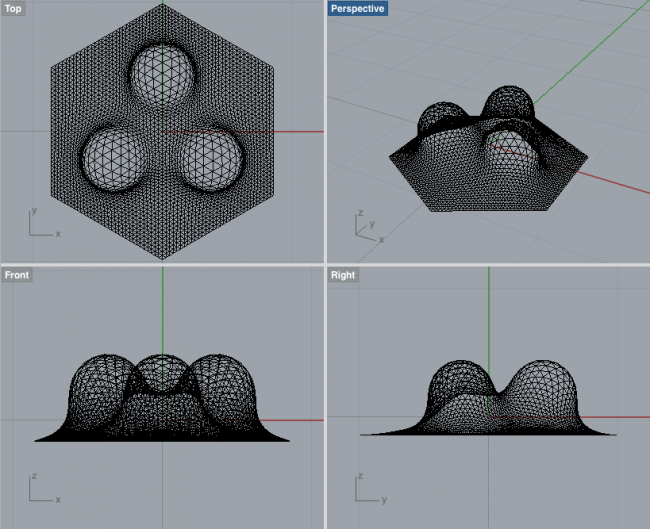This is my processing code (done inside Eclipse):

``````import processing.core.PApplet;
import toxi.geom.Vec3D;
import toxi.geom.mesh.TriangleMesh;
import toxi.processing.ToxiclibsSupport;

public class Main extends PApplet {
public static void main(String[] args) {
PApplet.main(new String[] { "Main" });
}

ToxiclibsSupport gfx;
TriangleMesh mesh;

public void setup()
{
size( 800, 600, P3D);
gfx = new ToxiclibsSupport(this);
}

public void draw()
{
background(151);
lights();
translate(width/2, height/2, 300);
rotateX(mouseY*0.01f);
rotateY(mouseX*0.01f);
gfx.origin(new Vec3D(), 100);
noStroke();
gfx.mesh(mesh, true, 10);
}
}
``````

This is what I see when I run the sketch: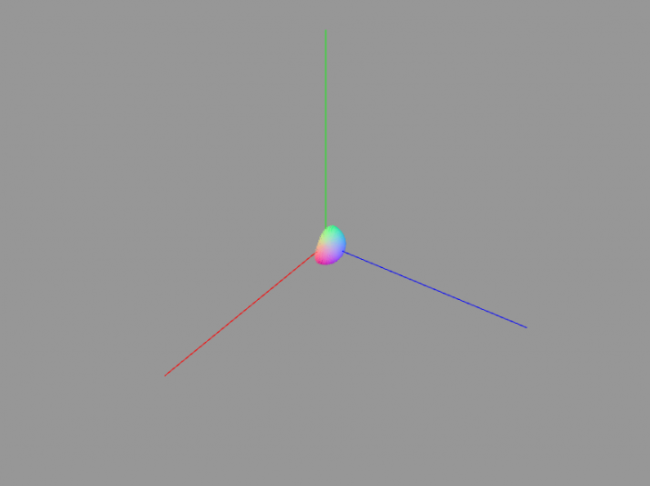• Hemesh & HemeshGUI errors

As far as I know Hemesh does not have such an import option. However, Toxiclibs does have an STLReader and Hemesh has a HEC_FromToxiTriangleMesh creator. So indirectly, you may be able to do it: STL > Toxiclibs TriangleMesh > Hemesh HE_Mesh.

Also check out this post: http://leonnicholls.com/hemesh-colorizer/

I think Leon used the approach decribed above and his code is open source.